# 5.1: The Unit Circle

•• Contributed by CK12
• CK12

The unit circle is a circle of radius one, centered at the origin, that summarizes all the $$30-60-90$$ and $$45-45-90$$ triangle relationships that exist. When memorized, it is extremely useful for evaluating expressions like $$\cos \left(135^{\circ}\right)$$ or $$\sin \left(-\frac{5 \pi}{3}\right)$$. It also helps to produce the parent graphs of sine and cosine.

How can you use the unit circle to evaluate $$\cos \left(135^{\circ}\right)$$ and $$\sin \left(-\frac{5 \pi}{3}\right) ?$$

### The Unit Circle

You already know how to translate between degrees and radians and the triangle ratios for 30-60-90 and 45-45-90 right triangles.  In order to be ready to completely fill in and memorize a unit circle, two triangles need to be worked out.  Start by finding the side lengths of a 30-60-90 triangle and a 45-45-90 triangle each with hypotenuse equal to 1.

$$\begin{array}{|l|l|l|} \hline 30^{\circ} & 60^{\circ} & 90^{\circ} \\ \hline x & x \sqrt{3} & 2 x \\ \hline \frac{1}{2} & \frac{\sqrt{3}}{2} & 1 \\ \hline \end{array}$$

$$\begin{array}{|l|l|l|} \hline 45^{\circ} & 45^{\circ} & 90^{\circ} \\ \hline x & x & x \sqrt{2} \\ \hline \frac{\sqrt{2}}{2} & \frac{\sqrt{2}}{2} & 1 \\ \hline \end{array}$$

This is enough information to fill out the important points in the first quadrant of the unit circle. The values of the $$x$$ and $$y$$ coordinates for each of the key points are shown below. Remember that the $$x$$ and $$y$$ coordinates come from the lengths of the legs of the special right triangles, as shown specifically for the $$30^{\circ}$$ angle. Always remember to measure the angle from the positive portion of the $$x$$ -axis.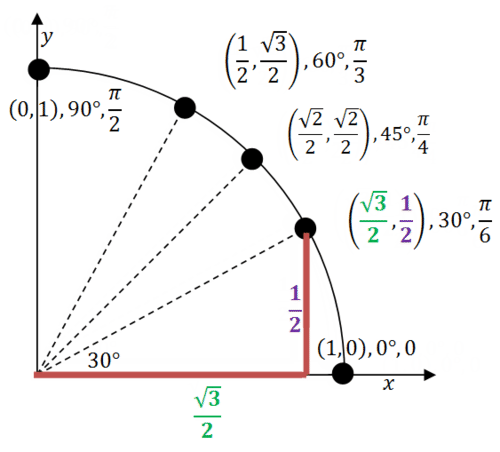Knowing the first quadrant well is the key to knowing the entire unit circle.  Every other point on the unit circle can be found using logic and this quadrant, so there is no need to memorize the whole circle.

To use your knowledge of the first quadrant of the unit circle to identify the angles and important points of the second quadrant, notice that the heights are mirrored and equal which correspond to the $$y$$ values. The $$x$$ values are all negative.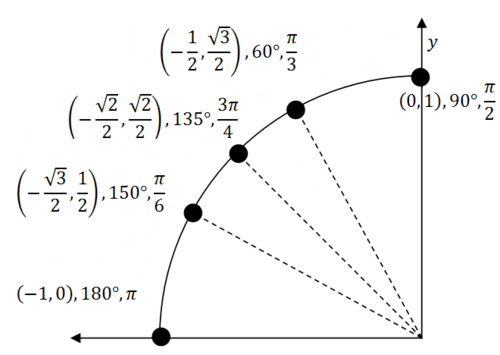There is a pattern in the heights of the points in the first quadrant that can help you remember the points.

Notice that the heights of the points in the first quadrant are the $$y$$ -coordinates: $$0, \frac{1}{2}, \frac{\sqrt{2}}{2}, \frac{\sqrt{3}}{2}, 1$$

When rewritten, the pattern becomes clear: $$\frac{0}{2}, \frac{\sqrt{1}}{2}, \frac{\sqrt{2}}{2}, \frac{\sqrt{3}}{2}, \frac{\sqrt{4}}{2}$$

The three points in the middle are the most often mixed up. This pattern illustrates how they increase in size from small $$\frac{1}{2},$$ to medium $$\frac{\sqrt{2}}{2},$$ to large $$\frac{\sqrt{3}}{2} .$$ When you fill in the unit circle, look for the heights that are small, medium and large and this will tell you were each value should go. Notice that the heights for these five points in the second quadrant are also $$0, \frac{1}{2}, \frac{\sqrt{2}}{2}, \frac{\sqrt{3}}{2}, 1$$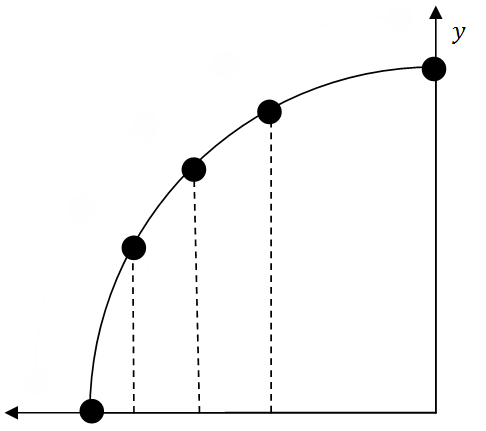This technique also works for the widths. This can make memorizing the 16 points of the unit circle a matter of logic and the pattern: $$\frac{0}{2}, \frac{\sqrt{1}}{2}, \frac{\sqrt{2}}{2}, \frac{\sqrt{3}}{2}, \frac{\sqrt{4}}{2}$$

One last item to note is that coterminal angles are sets of angles such as $$90^{\circ}, 450^{\circ},$$ and $$-270^{\circ}$$ that start at the positive x-axis and end at the same terminal side. since coterminal angles end at identical points along the unit circle, trigonometric expressions involving coterminal angles are equivalent: $$\sin (90)=\sin (450)=\sin (-270)$$

### Examples

Example 1

Earlier, you were asked how you can use the unit circle to evaluate $$\cos (135)$$ and $$\sin \left(-\frac{5 \pi}{3}\right)$$. The $$x$$ value of a point along the unit circle corresponds to the cosine of the angle. The $$y$$ value of a point corresponds to the sine of the angle. When the angles and points are memorized, simply recall the $$x$$ or $$y$$ coordinate. If the construction of the unit circle is understood, it becomes easier to determine the coordinates.

When evaluating $$\cos \left(135^{\circ}\right)$$ your thought process should be something like this:

You know $$135^{\circ}$$ goes with the point $$\left(-\frac{\sqrt{2}}{2}, \frac{\sqrt{2}}{2}\right)$$ and cosine is the $$x$$ portion. So, $$\cos \left(135^{\circ}\right)=-\frac{\sqrt{2}}{2}$$

$$\cos \left(135^{\circ}\right)=-\frac{\sqrt{2}}{2}$$

When evaluating $$\sin \left(-\frac{5 \pi}{3}\right)$$ your thought process should be something like this:

You know $$-\frac{5 \pi}{3}$$ goes with the point $$\left(\frac{1}{2},-\frac{\sqrt{3}}{2}\right)$$ and sine is the $$y$$ portion. So, $$\sin \left(-\frac{5 \pi}{3}\right)=-\frac{\sqrt{3}}{2}$$

Example 2

Evaluate $$\cos 60^{\circ}$$ using the unit circle and right triangle trigonometry. What is the connection between the $$x$$ coordinate of the point and the cosine of the angle?

The point on the unit circle for $$60^{\circ}$$ is $$\left(\frac{1}{2}, \frac{\sqrt{3}}{2}\right)$$ and the point is one unit from the origin. This can be represented as a $$30-60-90$$ triangle.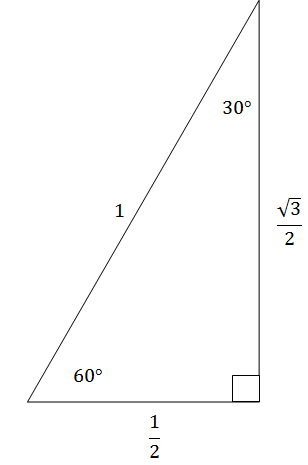Since cosine is adjacent over hypotenuse, cosine turns out to be exactly the $$x$$ coordinate $$\frac{1}{2}$$.

Example 3

Using knowledge of the first quadrant of the unit circle, identify the angles and important points of the third quadrant.

Both the $$x$$ values and $$y$$ values are negative and their respective coordinates correspond to those of the other quadrants.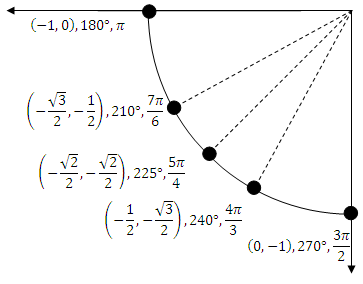Example 4

For each of the six trigonometric functions, identify the quadrants where they are positive and the quadrants where they are negative.

In quadrant I, the hypotenuse, adjacent and opposite side are all positive.  Thus all 6 trigonometric functions are positive.

In quadrant II the hypotenuse and opposite sides are positive and the adjacent side is negative.  This means that every trigonometric expression involving an adjacent side is negative.  Sine and its reciprocal cosecant are the only two trigonometric functions that do not refer to the adjacent side which makes them the only positive ones.

In quadrant III only the hypotenuse is positive.  Thus the only trigonometric functions that are positive are tangent and its reciprocal cotangent because these functions refer to both adjacent and opposite sides which will both be negative.

In quadrant IV the hypotenuse and the adjacent sides are positive while the opposite side is negative.  This means that only cosine and its reciprocal secant are positive.

A mnemonic device to remember which trigonometric functions are positive and which trigonometric functions are negative is “All Students Take Calculus.”  All refers to all the trigonometric functions are positive in quadrant I.  The letter S refers to sine and its reciprocal cosecant that are positive in quadrant II.  The letter T refers to tangent and its reciprocal cotangent that are positive in quadrant III.  The letter C refers to cosine and its reciprocal secant that are positive in quadrant IV.

Example 5

Evaluate the following trigonometric expressions using the unit circle.

1. $$\sin \frac{\pi}{2}$$

$$\sin \frac{\pi}{2}=1$$

2. $$\cos 210^{\circ}$$

$$\cos 210^{\circ}=-\frac{\sqrt{3}}{2}$$

3. $$\tan 315^{\circ}$$

$$\tan 315^{\circ}=-1$$

4. $$\cot 270^{\circ}$$

$$\cot 270^{\circ}=0$$

5. $$\sec \frac{11 \pi}{6}$$

$$\sec \frac{11 \pi}{6}=\frac{1}{\cos \frac{11 \pi}{6}}=\frac{2}{\sqrt{3}}=\frac{2 \sqrt{3}}{3}$$

6. $$\csc -\frac{5 \pi}{4}$$

$$\csc -\frac{5 \pi}{4}=\frac{1}{\sin -\frac{5 \pi}{4}}=-\frac{2}{\sqrt{2}}=-\sqrt{2}$$

Review

Name the angle between $$0^{\circ}$$ and $$360^{\circ}$$ that is coterminal with...

1. $$-20^{\circ}$$

2. $$475^{\circ}$$

3. $$-220^{\circ}$$

4. $$690^{\circ}$$

5. $$-45^{\circ}$$

Use your knowledge of the unit circle to help determine whether each of the following trigonometric expressions is positive or negative.

6. $$\tan 143^{\circ}$$

7. $$\cos \frac{\pi}{3}$$

8. $$\sin 362^{\circ}$$

9. $$\csc \frac{3 \pi}{4}$$

Use your knowledge of the unit circle to evaluate each of the following trigonometric expressions.

10. $$\cos 120^{\circ}$$

11. $$\sec \frac{\pi}{3}$$

12. $$\tan 225^{\circ}$$

13. $$\cot 120^{\circ}$$

14. $$\sin \frac{11 \pi}{6}$$

15. $$\csc 240^{\circ}$$

16. Find $$\sin \theta$$ and $$\tan \theta$$ if $$\cos \theta=\frac{\sqrt{3}}{2}$$ and $$\cot \theta>0$$.

17. Find $$\cos \theta$$ and $$\tan \theta$$ if $$\sin \theta=-\frac{1}{2}$$ and $$\sec \theta<0$$.

18. Draw the complete unit circle (all four quadrants) and label the important points.